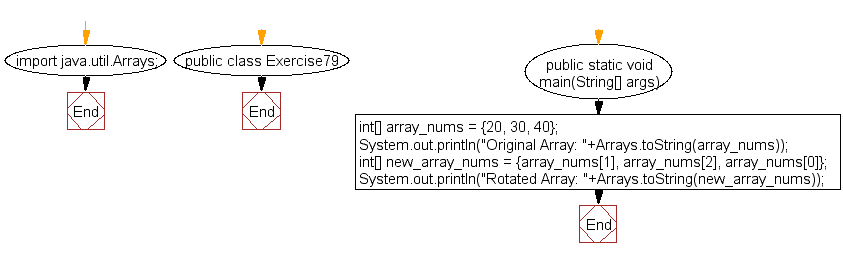﻿ Java exercises: Rotate an array of integers in left direction - w3resource# Java Exercises: Rotate an array of integers in left direction

## Java Basic: Exercise-79 with Solution

Write a Java program to rotate an array (length 3) of integers in left direction.
Test Data: {20, 30, 40}
Expected output: {30, 40, 20}

Sample Solution:

Java Code:

``````import java.util.Arrays;
public class Exercise79 {
public static void main(String[] args)
{
int[] array_nums = {20, 30, 40};
System.out.println("Original Array: "+Arrays.toString(array_nums));
int[] new_array_nums = {array_nums, array_nums, array_nums};
System.out.println("Rotated Array: "+Arrays.toString(new_array_nums));
}
}
```
```

Sample Output:

```Original Array: [20, 30, 40]
Rotated Array: [30, 40, 20]
```

Pictorial Presentation:Flowchart:Java Code Editor: